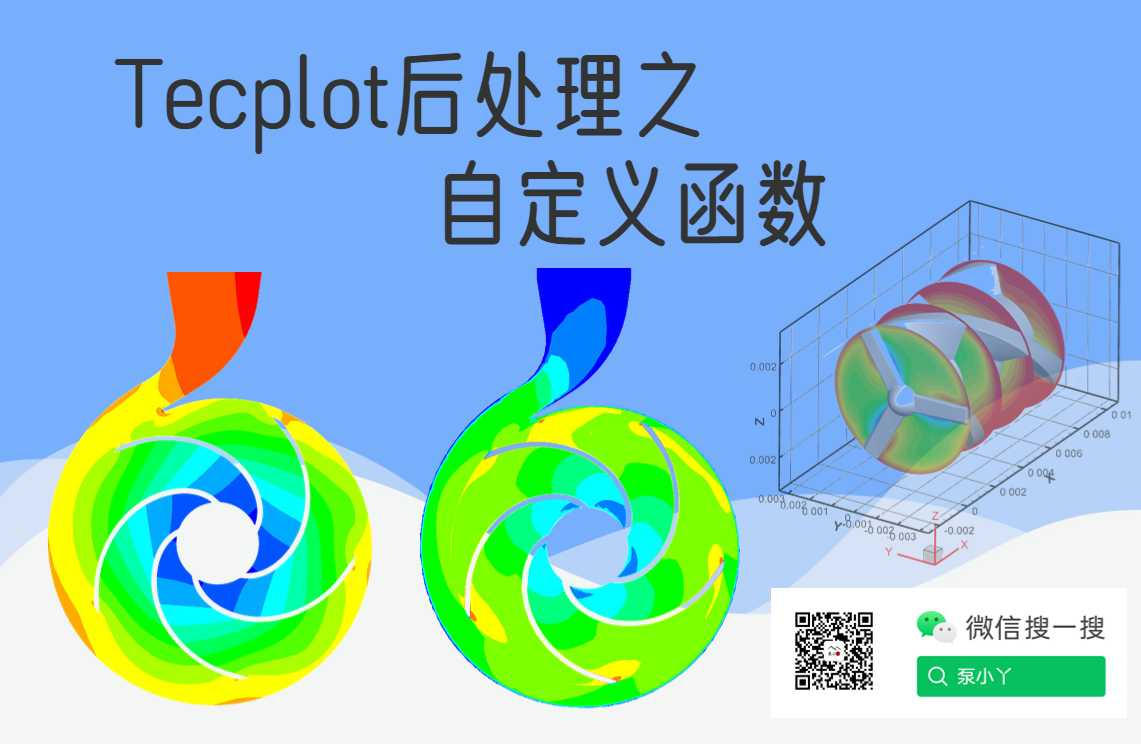# Tecplot后处理扩展工具：自定义函数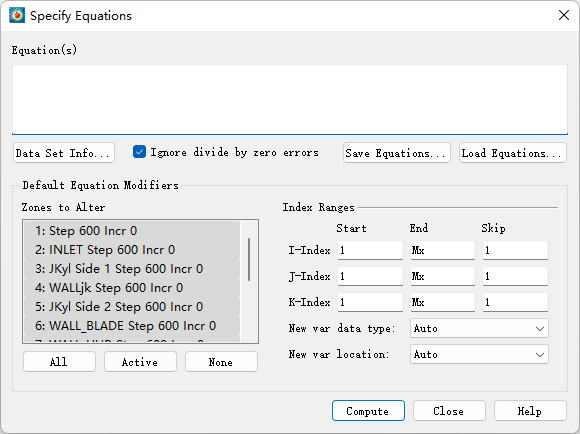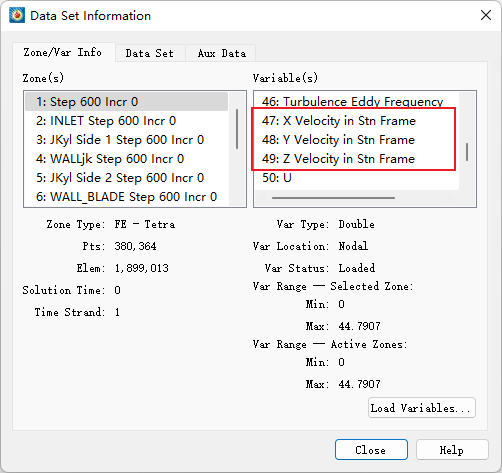{velocity}=sqrt(v47**2+v48**2+v49**2)

sqrt()是开根号的意思，v47**2的意思是计算v42也就是X方向速度的平方值，然后将3个方向上的速度平方值之和开根号就能计算出合速度。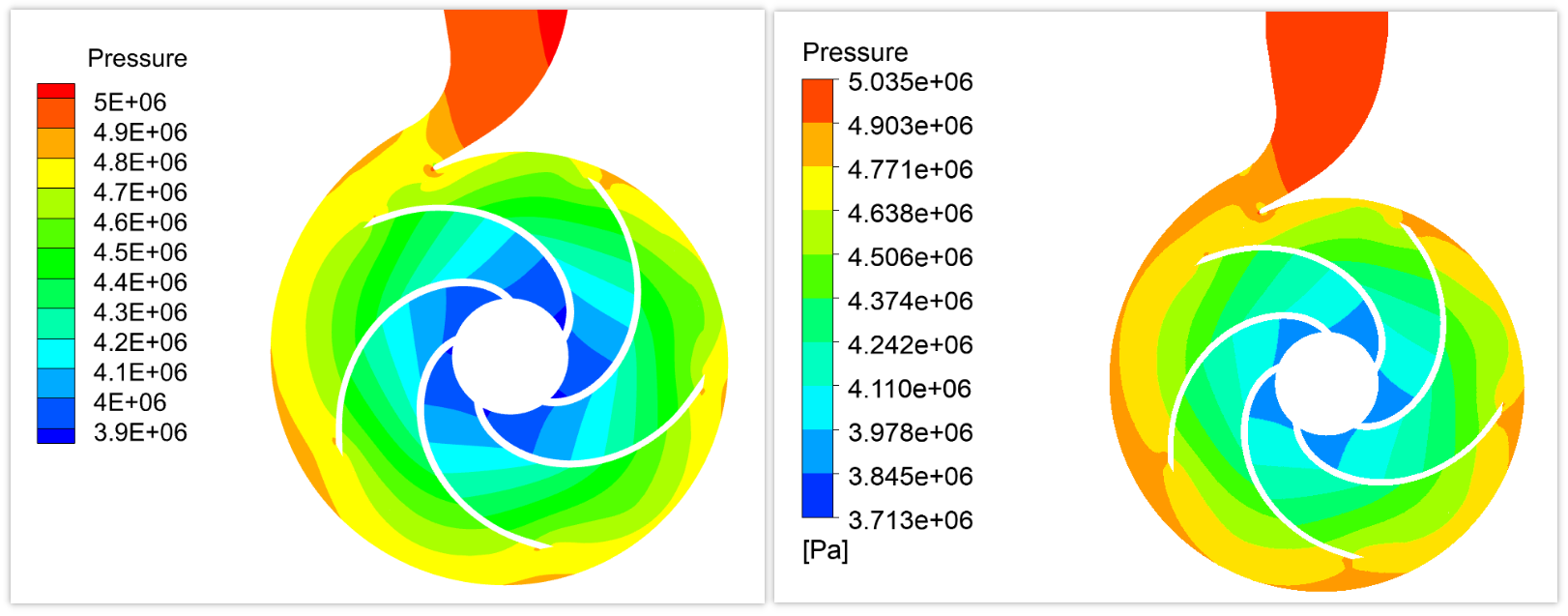Tecplot的公式功能还有很多用途，我们参照帮助文档中的说明来学习一波：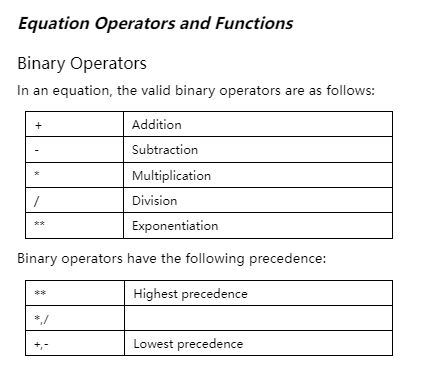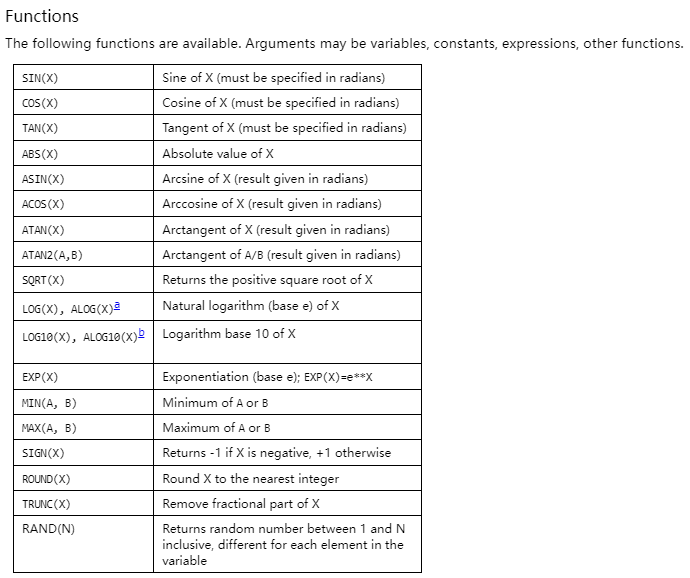条件表达式我们应该很少用到，如果是什么那么结果取什么，可以用于流场中筛选数据，格式为：IF(P,T,F)1、在以下等式中，V1（数据文件中定义的第一个变量）被 V1 现有值的两倍半替换：

V1 = 2.5*V1

2、以下等式将名为 Density 的变量的值设置为 205。如果名为 Density 的变量不存在，则会创建一个新变量。

{Density} = 205

3、在下一个等式中，Y（分配给 Y 轴的变量）的值被 X（分配给 X 轴的变量）的值平方的负数替换：

Y = -X**2

V3 = round(V2)

4、在以下等式中，数据集中第四个变量的值被第三个变量的值的对数（以 10 为底）替换。

V4 = ALOG10(V3)

5、假设第三个和第四个变量是速度的 X 和 Y 分量，并且目前总共有五个变量。以下示例创建了一个新变量 (V6)，它是速度分量的大小。

V6 = (V3*V3+V4*V4)**0.5

V6 = sqrt(V3**2+V4**2)

6、您还可以使用以下等式完成上述操作（假设您已经定义了活动框架的矢量分量）：

{Mag} = sqrt(U*U + V*V)

7、以下等式将名为 diff 的变量的值设置为从现有深度值中减去名为 depth 的变量的截断值：

{diff} = {depth} – trunc({depth})

8、在下一个等式中，假设 C 和 S 都已定义，则 C（轮廓变量）设置为 S（散布大小变量）的绝对值：

C = abs(S)

8、在以下示例中，创建了一个新变量（假设数据文件中最初只存在七个变量）。 V8（新变量）的值是根据现有变量的函数计算得出的：

V8 = SQRT((V1*V1+V2*V2+V3*V3)/(287.0*V4*V6))

9、上述操作可以通过两个更简单的步骤执行，如下所示：

V8 = V1*V1+V2*V2+V3*V3
V8 = SQRT(V8/(287.0*V4*V6))

10、以下等式将名为 TIME 的任何低于 5.0 的变量值替换为 5.0。换句话说，TIME 的值被替换为 TIME 的当前值和 5.0 中的最大值：

{TIME} = max({TIME},5)

11、以下等式创建变量 V4，其在 X<0 的点处具有 X 值；在其他点，它的值为零（这不会影响 X 的任何值）：

V4 = min(X,0)

12、另一个使用内部函数的示例如下所示：

V8 = 55.0*SIN(V3*3.14/180.0) + ALOG(V4**3/(v1+1.0))

13、您还可以在方程式中引用 I、J 和 K 指数。例如，如果您想使用值消隐来切出一个区域的一部分，您可以创建一个新变量，它是 I 和 J 指数的函数（用于 IJ 有序数据）。然后，通过使用值消隐，您可以删除值消隐变量的值小于或等于值消隐截止值的某些单元格。

14、下面是一个计算值消隐变量的示例，该变量在 I=10 到 30 的单元格块中为零，在其他单元格中等于 1：

V3 = min((max(I,30)-min(I,10)-20),1)

15、以下等式将 Y 的所有值替换为 Y 的当前值与区域 1 中的 Y 值之间的差值。（如果区域 1 用于数据更改，则 Y 的新值将在该区域中为零。）

Y = Y – Y

16、以下等式将 V3 的值（在 IJ 有序区域中）替换为四个相邻数据点的 V3 值的平均值：

V3 = (V3(i+1,j)+V3(i-1,j)+V3(i,j+1)+V3(i,j-1))/4

17、以下等式将名为 TEMP 的变量的值设置为在四个位置测量的名为 T 的变量的值的乘积：在区域 1 中，在当前数据点之前的两个索引值处，在当前区域中，在绝对索引处 3 ，在当前数据点的区域 4 中，在当前数据点的当前区域中。

{TEMP} = {T}(i-2) * {T}(3) * {T} * {T}

18、指数可以与区域规格相结合。首先列出区域，然后列出索引偏移量。例如：

V3 = V3 – V3(i+1)
Y = Y – Y(1) + Y(1,j+3) + Y

19、通过字母代码引用变量：

V3 = I + J
V4 = cos(X) * cos(Y) * cos(Z)
{距离} = sqrt(U*U + V*V + W*W)
{温度} = min(B,1)

20、为给定变量指定区域编号：

V3 = V3 – V3
X = ( X + X + X ) / 3
V8 = V1 – 2*C + {R/T}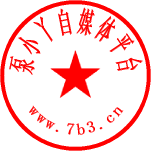## Fluent meshing中如何查看网格质量分布

2022-7-11 22:56:32

## Cfturbo导入TurboGrid的三种方法

2022-10-5 14:41:58

2 条回复 A文章作者 M管理员
1. 长知识了

2. 以前就会求个速度，了解了